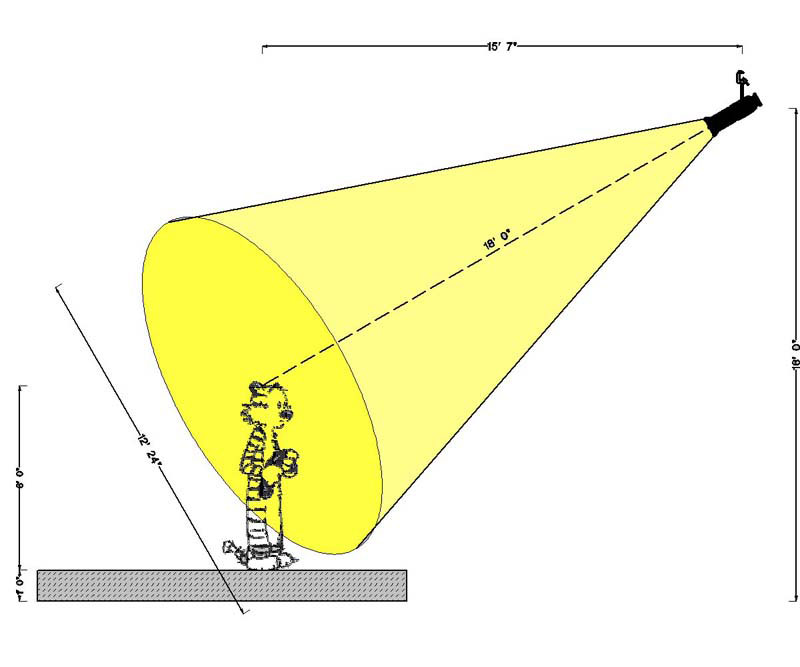MF
Multiplication Factors
or
How Do You Know Which Light to Use?

In addition to knowing specific features different light fixtures can provide the lighting designer (such as whether the light can offer a sharp edged pool of light, or whether it accepts a gobo pattern, or can the light be shuttered off unwanted areas), the designer needs to know the size of pool of light specific lighting instruments provide.

Designers determine the size of the pool of light for specific fixtures by using the MF factor provided by the manufacturer. Usually a decimal number, the MF factor is multiplied by the distance the fixture is hung from the lighting area to determine the size of pool of light.

S = MF x D

"S" is the size of pool of light.
"D" is the distance of the fixture from the lighting area.
"MF" is the multiplication factor provided by the manufacturer.

In the example below, the throw is 18'-0" and the fixture's MF is .68, giving a pool of light 12'-3".

(18 x 0.68 = 12.24)from Stage Lighting for Students
by Jeffrey E. Salzberg
with Judy Kupferman

Designers use a vertical section of the performance venue to determine the distance each fixture will be from each lighting area. Using a calculator and the MF factor, the size of the pool of light can be determined.

Designers use this same formula to solve a number of problems presented by a specific design. If a designer knows where they want to hang a fixture, as well as the preferred size of the pool of light, they can use the following variation of the formula to determine the most ideal fixture for a specific location.

MF = S divided by D

If the designer wants to know how far away a fixture should be hung from an area being lit by a specific lighting instrument (which is often the case when determining how high to trim on-stage electrics), the formula could be altered as follows to determine the distance the fixture should be from the lighting area, which is 6'-0" above the stage floor.

D = S divided by MF

In all cases, designers use the MF factor to calculate:

• Which fixture to use.
• Where to hang the fixture.
• Correct size of pool of light on-stage.

This process eliminates guesswork that might otherwise be necessary.